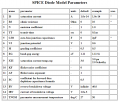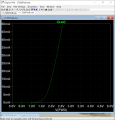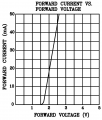# The most simple LTSpice LED simulation question

#### Lucky-Luka

Joined Mar 28, 2019
174
Hi all
I just wanted to simulate a 5mm green LED connected to a DC source.
I expected to see more than 2V at the A point of the circuit but it looks like it's more or less 0.7V which is the typical forward voltage of a diode.
Where i am going wrong?
Thanks

#### Attachments

• 723 bytes Views: 13
• 41.9 KB Views: 34

#### dl324

Joined Mar 30, 2015
15,405
Where i am going wrong?
It's your model. You specified a junction voltage of 0.75V.

#### Lucky-Luka

Joined Mar 28, 2019
174
It's your model. You specified a junction voltage of 0.75V.
There are others parameters too... This model should represent a 2.1V LED and the parameter N=4.61 should count something. No?

#### Lucky-Luka

Joined Mar 28, 2019
174
hi LL,
Try this Gn LED.
E
Thanks
I have found that model and I thought it was ok.

#### Jony130

Joined Feb 17, 2009
5,435
Where i am going wrong?
Simply change the LED2 to D1 and D to LED2 and everything will work.
Do you see your mistake now?

#### Attachments

• 738 bytes Views: 6
• 25.2 KB Views: 18
•Lucky-Luka

#### Lucky-Luka

Joined Mar 28, 2019
174
Simply change the LED2 to D1 and D to LED2 and everything will work.
Do you see your mistake now?
I see.
Thanks

#### k1ng 1337

Joined Sep 11, 2020
701
Give falstad.com/circuit/ simulator a try if you haven't already. I like how many of the examples can be used as templates for your own circuits and it's GUI is a lot easier than spice if your having some trouble.

#### dl324

Joined Mar 30, 2015
15,405
There are others parameters too... This model should represent a 2.1V LED and the parameter N=4.61 should count something. No?
I'm not much of a LTspice user. I prefer to do the vast majority my simulations in my head.

From https://www.acsu.buffalo.edu/~wie/applet/spice_pndiode/spice_diode_table.htmlA value of 4.61 seems unreasonable to me.

#### ericgibbs

Joined Jan 29, 2010
16,741
hi dl,
The N parameter for LED's is higher than that for regular Diodes.
Clip from LTS standard.dio folder.

eg;
.MODEL LedRed D (IS=93.2P RS=42M N=3.73 BV=4 IBV=10U CJO=2.97P VJ=.75 M=.333 TT=4.32U Iave=40m Vpk=4 type=LED)
.MODEL LedGreen D (IS=93.2P RS=42M N=4.61 EG=4 BV=4 IBV=10U CJO=2.97P VJ=.75 M=.333 TT=4.32U Iave=40m Vpk=4 type=LED)
.MODEL LedBLUE D (IS=93.2P RS=42M N=7.47 BV=5 IBV=10U CJO=2.97P VJ=.75 M=.333 TT=4.32U Iave=40m Vpk=5 type=LED)
.model LedWHITE D(Is=0.27n Rs=5.65 N=6.79 Cjo=42p Iave=30m Vpk=5 type=LED)
.model BLUE D(Is=25e-14 N=5 Rs=14 Eg=2.23 Tnom=27deg type=LED)
.model GREEN D(Is=1e-19 N=2 Rs=1.5 Eg=2.23 Tnom=27deg type=LED)
.model RED D(Is=1.0p N=3 Rs=9 Eg=2.17 Tnom=27deg type=LED)

•Lucky-Luka

#### dl324

Joined Mar 30, 2015
15,405
The N parameter for LED's is higher than that for regular Diodes.
It's a don't care for me. I rarely use LTspice, or any other analog simulator, and I don't think I'll ever need a detailed simulation for an LED.

#### eetech00

Joined Jun 8, 2013
3,418
hi dl,
The N parameter for LED's is higher than that for regular Diodes.
Clip from LTS standard.dio folder.

eg;
.MODEL LedRed D (IS=93.2P RS=42M N=3.73 BV=4 IBV=10U CJO=2.97P VJ=.75 M=.333 TT=4.32U Iave=40m Vpk=4 type=LED)
.MODEL LedGreen D (IS=93.2P RS=42M N=4.61 EG=4 BV=4 IBV=10U CJO=2.97P VJ=.75 M=.333 TT=4.32U Iave=40m Vpk=4 type=LED)
.MODEL LedBLUE D (IS=93.2P RS=42M N=7.47 BV=5 IBV=10U CJO=2.97P VJ=.75 M=.333 TT=4.32U Iave=40m Vpk=5 type=LED)
.model LedWHITE D(Is=0.27n Rs=5.65 N=6.79 Cjo=42p Iave=30m Vpk=5 type=LED)
.model BLUE D(Is=25e-14 N=5 Rs=14 Eg=2.23 Tnom=27deg type=LED)
.model GREEN D(Is=1e-19 N=2 Rs=1.5 Eg=2.23 Tnom=27deg type=LED)
.model RED D(Is=1.0p N=3 Rs=9 Eg=2.17 Tnom=27deg type=LED)
There is no LEDs like that in the standard.dio distribution.

#### eetech00

Joined Jun 8, 2013
3,418
Hi all
I just wanted to simulate a 5mm green LED connected to a DC source.
I expected to see more than 2V at the A point of the circuit but it looks like it's more or less 0.7V which is the typical forward voltage of a diode.
Where i am going wrong?
Thanks
Try this LED L200TGB5:
* Hi-Eff Green, GAP/GAP
.Model L200TG5B D(IS=1.69111e-008 N=5.23202 RS=11.3667 CJO=53p BV=5.0 IBV=100u IAVE=30m mfg=LEDTRONICS type=LED)

Its a better LED model.•Lucky-Luka